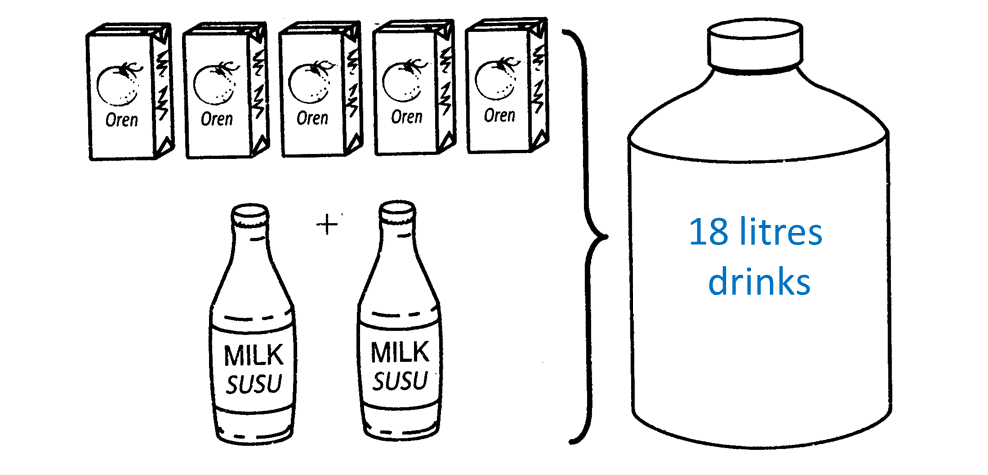11.2.2 Linear Equations II, PT3 Focus Practice

Question 6:
Diagram below shows 5 boxes of orange juice and 2 bottles of milk. Both orange juice and milk are mixed to produce 18 litres drink.(a) Based on the above situation, write a linear equation.
(b) If 9 litres of drinks is produced by using 2 boxes of orange juice and 2 bottles of milk, find the volume, in litre, of orange juice in each box.

Solution:
Let j be the number of boxes of orange juice and m be the number of bottles of milk.
(a)
5j + 2m = 18

(b)